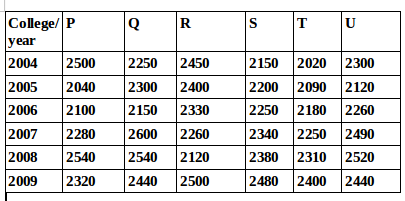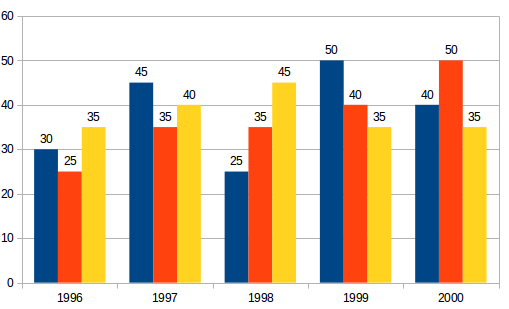## Data Interpretation For SBI PO : Set – 59

D.1-5) Study the following table carefully to answer the questions that follow:

Number of students studying in six different colleges over the years1) What is the total number of students from all the colleges together in the year 2005?

a) 10350

b) 13150

c) 15310

d) 11350

e) None of these

b)

Required number = 2040+2300+2400+2200+2090+2120=13150

2) What is the percent increase in the number of students in College T in the year 2007 from the previous year?

a) 8.33%

b) 5.18%

c) 6.63%

d) 3.21%

e) None of these

d)

Required Percentage growth = (2250-2180)/2180 *100=3.21%

3) What is the ratio of the total number of student in College S in the years 2006 and 2009 together to the  total number of students in College U in the Same years?

a) 473:470

b) 470:473

c) 371: 390

d) 390:371

e) None of these

a)

Required Ratio = (2250+2480):(2260+2440)=4730:4700=473:470

4) What is the average number of students in all the colleges together in the year 2004?

a) 2208

b) 2196

c) 2144

d) 2324

e) 2278

e)

Required average number = (2500+2250+2450+2150+2020+2300/6)*100=13670/6=2278

5) The number of students in College P in the year 2008 forms approximately what percent of the total number of students in that college in all the years together?

a) 11%

b) 31%

c) 18%

d) 26%

e) 23%

c)

Required % = 2450/(2500+2040+2100+2280+2540+2320)*100=254000/13780=18.43=18%6) What is the difference between the production of company Z in 1998 and company Y in 1996?

a) 2,00,000 tons

b) 20,00,000 tons

c) 20,000 tons

d) 2,00,00,000 tons

e) None of these

b)

Required difference

= [(45 – 25) * 1,00,000] tones
= 20,00,000 tons

7) What is the ratio of the average production of company X in the period 1998-2000 to the average production of company Y in the same period?

a) 1:1

b) 15:17

c) 23:25

d) 27:29

e) None of these

c)

Average production of company X in the period 1998-2000 = [1/3 * (25 + 50 + 40)] = (115/3) lakh tons. Average production of company Y in the period 1998-2000 = [1/3 * (35 + 40 + 50)] = (125/3) lakh tons. Required ratio = (115/3)/(125/3) = 115/125 = 23/25

8) What is the percentage increase in the production of company Y from 1996 to 1999?

a) 30%

b) 45%

c) 50%

d) 60%

e) 75%

d)

Percentage increase in the production of company Y from 1996 to 1999

= [(40 – 25)/25 * 100]% = (15/25 * 100)% = 60%

9) The average production for five years was maximum for which company?

a) X

b) Y

c) Z

d) X and Y both

e) X and Z both

e)

For company

X = [1/5 * (30 + 45 + 25 + 50 + 40)] = 190/5 = 38

For company

Y = [1/5 * (25 + 35 + 35 + 50 + 40)] = 185/5 = 37

For company

Z = [1/5 * (35 + 40 + 45 + 35 + 35)] = 190/5 = 38 Average production of five years in maximum for both the companies X and Z.

10) In which year was the percentage of production of company Z to the production of company Y the maximum?

a) 1996

b) 1997

c) 1998

d) 1999

e) 2000

a)

The percentage of production of company Z to the production of company Z for various years are:

For 1996 = (35/25 * 100)% = 140%

For 1997 = (40/35 * 100)% = 114.29%

For 1998 = (45/35 * 100)% = 128.57%

For 1999 = (35/40 * 100)% = 87.5%

For 2000 = (35/50 * 100)% = 70%

Clearly, this percentage is highest for 1996.